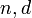# Burnside problem

Jump to: navigation, search

This article describes an open problem in the following area of/related to group theory: presentation theory

## Statement

For what values of$n$ are the following equivalent conditions true?

1. Every group of exponent$n$ is locally finite
2. Every finitely generated group of exponent$n$ is finite
3. For every positive integer$d$, the Burnside group$B(d,n)$ is a finite group.

Note that, at least prima facie, it is possible, for a given$n$, that$B(d,n)$ is finite for small$d$ but infinite for large$d$.

## Facts

### Small exponent cases

Value of$n$ Answer to Burnside's question Nilpotency class of$B(d,n)$ in terms of$n$ (assume$d \ge 1$) Order of$B(d,n)$ in terms of$n,d$ Explanation and comments
1 Yes 0 1 The only possible group is the trivial group
2 Yes 1$2^d$ exponent two implies abelian, so any group of exponent 2 must be an elementary abelian 2-group
3 Yes 1 for$d = 1$
2 for$d = 2$
3 for$d \ge 3$$3^r$ where$r = d + \binom{d}{2}+ \binom{d}{3}$ exponent three implies class three: this follows from exponent three implies 2-Engel for groups, 2-Engel implies class three for groups
4 Yes 1 for$d = 1$
PLACEHOLDER FOR INFORMATION TO BE FILLED IN: [SHOW MORE]
?
5 Unknown 1 for$d = 1$
Unknown for$d \ge 2$
?
6 Yes 1 for$d = 1$
Not nilpotent for$d \ge 2$
?

### Large exponent cases

Statement Best known bounds
For very large values of$n$, the group$B(d,n)$ is infinite for every$d > 1$. In particular,$B(2,n)$ is infinite. Definitely true for all primes greater than$10^{75}$, because of the existence of Tarski monsters. This property also holds for all odd$n$ greater than or equal to 665, and for all even$n$ greater than or equal to 8000.